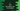# How to find the square root of a number in C## How to find the square root of a number in C:

In C, math.h header file containes important utility functions. There is one function called sqrt, which can be used to find the square root of a number in C. In this post, we will learn how to use sqrt method with definition and examples.

## Definition of sqrt:

sqrt is defined as like below:

``double sqrt(double num)``

It takes one double value as the parameter and returns the square root for that number. The return value is in double.

## Example of sqrt:

Let’s take a look at one example on how to use sqrt:

``````#include <stdio.h>
#include <math.h>

int main()
{
double num, sqrtNum;

printf("Enter a number: ");
scanf("%lf", &num);

sqrtNum = sqrt(num);

printf("Square root is : %.2lf", sqrtNum);

return 0;
}``````

Here,

• num and sqrtNum are two double variables.
• It is asking the user to enter a number. It reads that number as double using %lf and stores that in num.
• Using sqrt, it finds the square root of the number. This value is stored in sqrtNum. To use sqrt, we need to use math.h header file.
• The last printf is printing the square root calculated.

If you run this program, it will print output as like below:

``````Enter a number: 100
Square root is : 10.00

Enter a number: 111
Square root is : 10.54

Enter a number: 144
Square root is : 12.00``````

## Finding the square root of integer or float:

We can use this method to find the square root for any other data types like integer or float. We can explicitly convert the type using double().

For example,

``````#include <stdio.h>
#include <math.h>

int main()
{
int num, sqrtNum;

printf("Enter a number: ");
scanf("%d", &num);

sqrtNum = (int)sqrt((double)num);

printf("Square root is : %.2d", sqrtNum);

return 0;
}``````

In this example, we are reading the number as integer and storing it in num. We are passing the num to sqrt by converting it to double.

The return value of sqrt is converted to integer because the return value is a double.

If you run this program, it will print similar output.